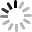# SVG D3.js - 座標軸 ( Axis )

## Axis 的 API

• axis.scale
• axis.orient
• axis.ticks
• axis.tickValues
• axis.tickSize
• axis.innerTickSize
• axis.outerTickSize
• axis.tickFormat

## axis.scale([scale])、axis.ticks([arguments…])

``````  var data = [
{x: 0,y: 1.89},
{x: 1,y: 2.77},
{x: 2,y: 0.86},
{x: 3,y: 3.45},
{x: 4,y: 4.13},
{x: 5,y: 3.59},
{x: 6,y: 2.33},
{x: 7,y: 3.79},
{x: 8,y: 2.61},
{x: 9,y: 2.15}
];

var width = 240,
height = 120;

var s = d3.select('#s');

s.attr({
'width': '300',
'height': '180'
}).style({
'border': '1px dotted #aaa'
});

var scaleX = d3.scale.linear()
.range([0, width])
.domain([0, 9]);

var scaleY = d3.scale.linear()
.range([height, 0])
.domain([0, 5]);

var line = d3.svg.line()
.x(function(d) {
return scaleX(d.x);
}).y(function(d) {
return scaleY(d.y);
});

var axisX = d3.svg.axis()
.scale(scaleX)
.ticks(10);

var axisY = d3.svg.axis()
.scale(scaleY)
.ticks(10);

s.append('path')
.attr({
'd': line(data),
'stroke': '#09c',
'fill': 'none'
});

s.append('g')
.call(axisX);  //call axisX

s.append('g')
.call(axisY);  //call axisY
``````## axis.orient([orientation])

``````  var axisX = d3.svg.axis()
.scale(scaleX)
.orient("bottom")
.ticks(10);

var axisY = d3.svg.axis()
.scale(scaleY)
.orient("left")
.ticks(10);
````````````  s.append('path')
.attr({
'd': line(data),
'stroke': '#09c',
'fill': 'none',
'transform':'translate(35,20)'  //折線圖也要套用 translate
});

s.append('g')
.call(axisX)
.attr({
'fill':'none',
'stroke':'#000',
'transform':'translate(35,'+(height+20)+')'
});

s.append('g')
.call(axisY)
.attr({
'fill':'none',
'stroke':'#000',
'transform':'translate(35,20)'
});
````````````  s.append('g')
.call(axisX)
.attr({
'fill':'none',
'stroke':'#000',
'transform':'translate(35,'+(height+20)+')'
}).selectAll('text')
.attr({
'fill':'#000',
'stroke':'none',
}).style({
'font-size':'11px'
});

s.append('g')
.call(axisY)
.attr({
'fill':'none',
'stroke':'#000',
'transform':'translate(35,20)'
}).selectAll('text')
.attr({
'fill':'#000',
'stroke':'none',
}).style({
'font-size':'10px'
});
``````## axis.tickFormat([format])

``````  var axisX = d3.svg.axis()
.scale(scaleX)
.orient("bottom")
.ticks(10)
.tickFormat(function(d){return d+'n';});

var axisY = d3.svg.axis()
.scale(scaleY)
.orient("left")
.ticks(5)
.tickFormat(function(d){return d+'%';});
````````````  var axisX = d3.svg.axis()
.scale(scaleX)
.orient("bottom")
.ticks(10)
.tickFormat(d3.format(",%"));
``````## axis.tickSize([inner, outer])

tickSize 表達的是座標軸上刻度線條的尺寸，包含了內部線段和和最外邊的線段，共兩個數值，而這兩個數值也各自獨立出來，所以其實直接用 tickSize 就可以都滿足，座標軸上預設的刻度長度是 6 ，數字負值往座標內縮，正值往座標外長出去，利用 tickSize，我們可以輕鬆地做出座標的內格線 ( Grid ) ，做法就是新增另外兩組 g 來放座標格線的 axis，然後讓刻度尺寸長度和圖表寬高一樣就可以，而且這裡頭使用剛剛講的 tickFormat("")，就不會有額外的座標標籤產生。( 範例：svg-d3-04-axis-demo5.html )

``````  var axisXGrid = d3.svg.axis()
.scale(scaleX)
.orient("bottom")
.ticks(10)
.tickFormat("")
.tickSize(-height,0);

var axisYGrid = d3.svg.axis()
.scale(scaleY)
.orient("left")
.ticks(10)
.tickFormat("")
.tickSize(-width,0);

s.append('g')
.call(axisXGrid)
.attr({
'fill':'none',
'stroke':'rgba(0,0,0,.1)',
'transform':'translate(35,'+(height+20)+')'
});

s.append('g')
.call(axisYGrid)
.attr({
'fill':'none',
'stroke':'rgba(0,0,0,.1)',
'transform':'translate(35,20)'
});
``````## axis.tickValues([values])

``````  var axisX = d3.svg.axis()
.scale(scaleX)
.orient("bottom")
.tickValues([1,3,5,7,9])
.tickFormat(function(d){return d+'n';});
```````````` var axisX = d3.svg.axis()
.scale(scaleX)
.orient("bottom")
.tickValues([1,2,6,7,9])
.tickFormat(function(d){return d+'n';});
````````````  var axisX = d3.svg.axis()
.scale(scaleX)
.orient("bottom")
.tickValues([1,3,5,7,9])
.tickFormat(function(d){return d+'n';})

var axisY = d3.svg.axis()
.scale(scaleY)
.orient("left")
.ticks(5)
.tickFormat(function(d){return d+'%';})